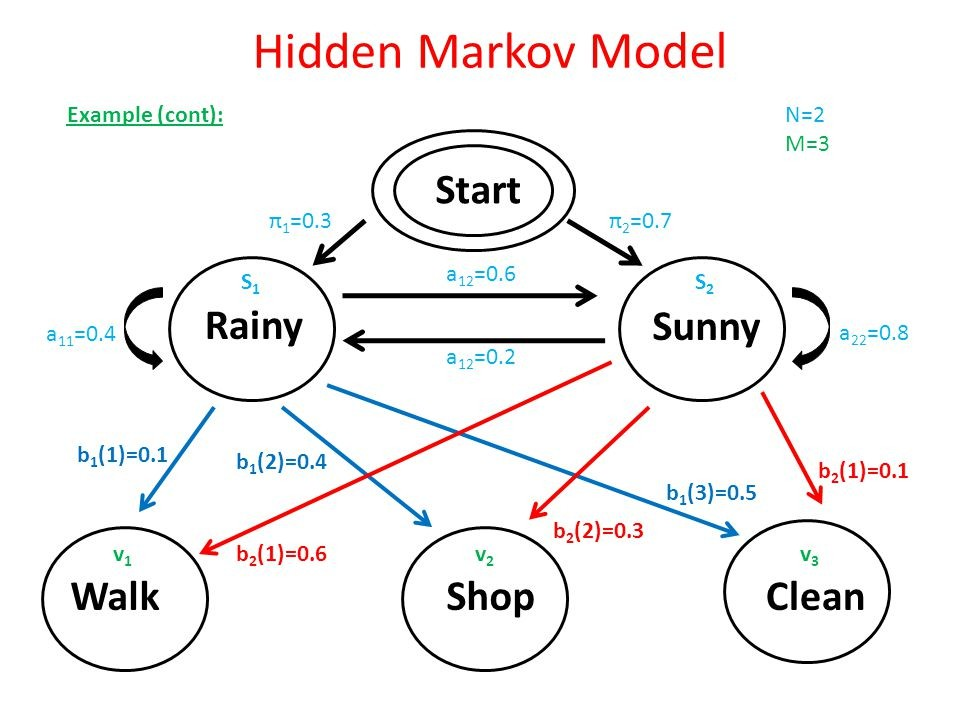## Markov and Hidden Markov Models

Pattern recognition is of interest in several fields, and Markov models offer a particularly powerful technique for solving such problems. Hidden Markov models (HMMs) go a step further, and can paradoxically sometimes be used to find patterns even when it is not entirely clear as to what one is looking for. In this article we introduce the basic ideas behind these models and illustrate them through applications to examples. We construct a Markov model for distinguishing between two languages which use a common script (as a prototypical problem, with straightforward applications to analyzing biological sequences, say), and a Hidden Markov model for detecting whether or not a fair coin was used in a coin toss (as a means of uncovering match fixing, for instance).

The use of Markov models and HMMs in pattern recognition is best described through examples and applications, and in this article, we will try to show how such analysis can be used in order to detect patterns in symbol strings. The symbol strings we will deal with could arise from languages (such as English or Italian), from biological macromolecules

(such as DNA or RNA) or from games of chance, such as the throw of dice or the toss of coins.Markov models are extensively used in bioinformatics to analyze DNA, RNA and protein sequences. Currently, these provide the most powerful methods of finding genes as well as other biologically important features such as promoter elements, CpG islands, etc. In the next two Sections we construct a model that can be taught to distinguish between English and Italian. In Section 4 we discuss Hidden Markov models and some of the standard algorithms used. The implementation we discuss is regrettably current: How can one figure out whether a (hypothetical) cricket match is fixed or played fairly

Conclusion

The use of Markov models and HMMs in pattern recognition is best described through examples and applications, and in this article, we tried to show how such analysis can be used in order to detect patterns in symbol strings. Hidden Markov models were originally developed in the field of speech recognition. These can be adapted and applied to more difficult pattern recognition problems. Thus Markov models and HMMs may help emerge new, highly efficient and robust Pattern Recognition Techniques.

1 reviews
•Markov and Hidden Markov Models

2 years ago